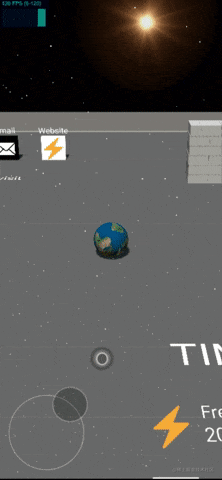# 用Three.js打造酷炫3D个人网站（含源码）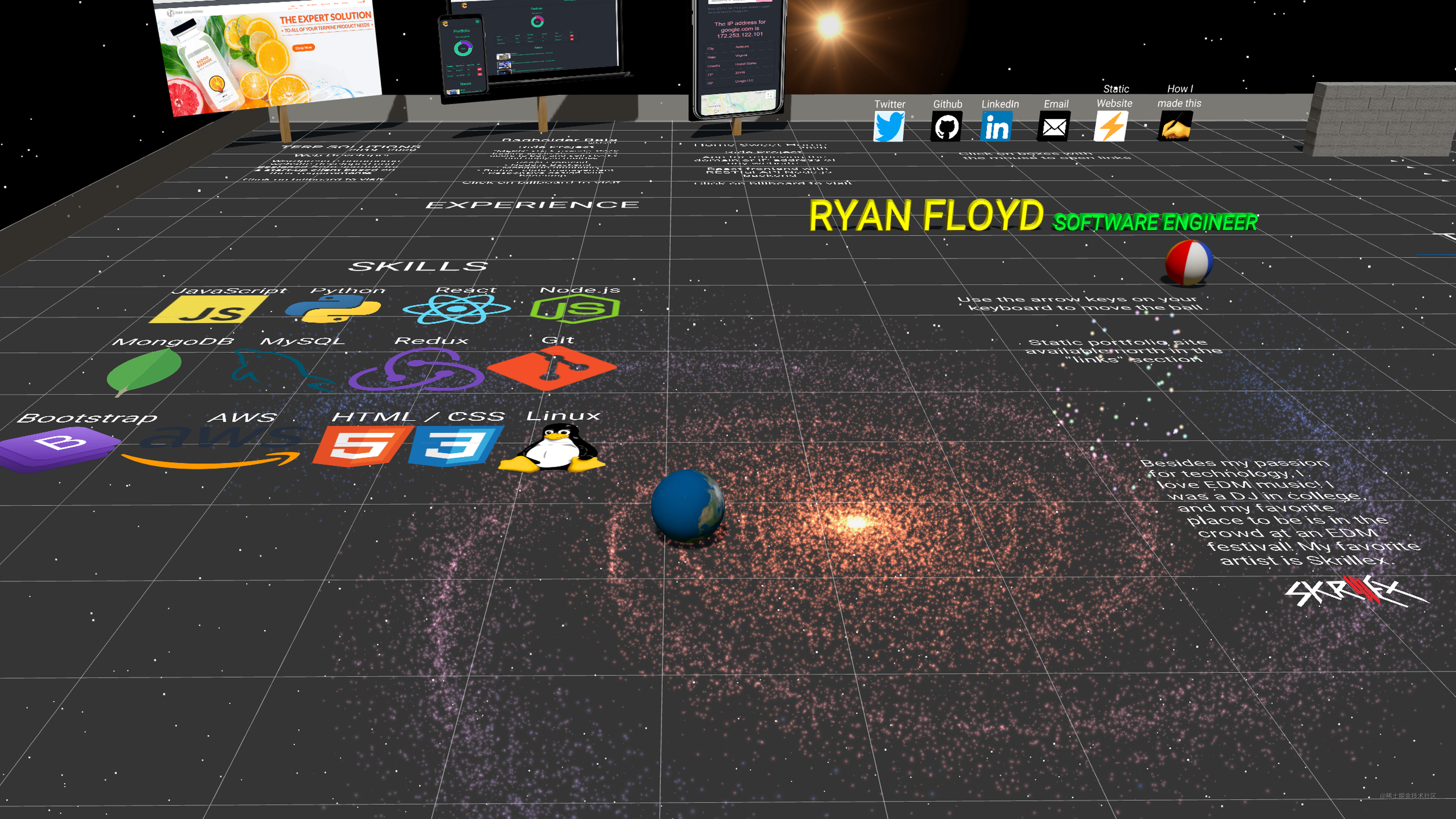# 引言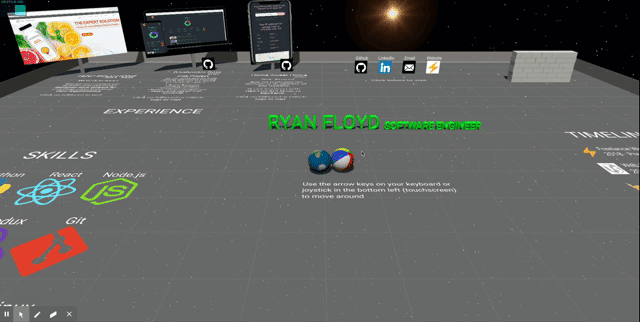# Three.js的3D世界

`three.js`是一个让3D网页应用开发变得简单的库。它诞生于2010年，作者是Ricardo Cabello (Mr.doob),，在github上有超过1300多的贡献者，在所有仓库中star数排行第38。

# Three.js的工作机制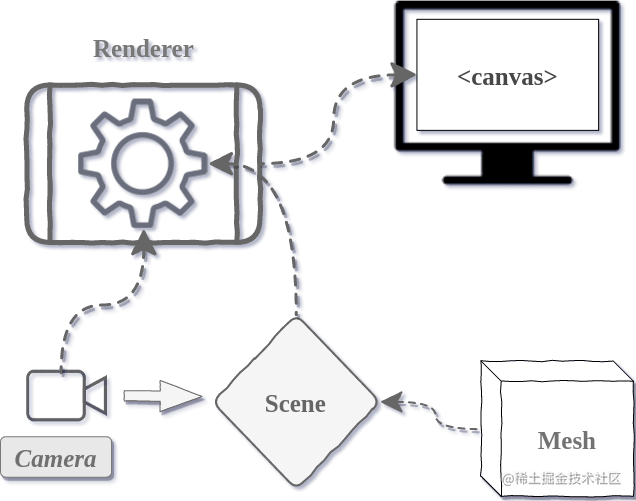(3D应用的组件结构，图片来自discoverthreejs.com)

`Three.js`使得在浏览器展示3D图像变得容易，它的底层是基于`WebGL`，它使浏览器能借助系统显卡在canvas中绘制3D画面。

`WebGL`自身只能绘制点（points）、线（lines）和三角形（triangles），而`Three.js``WebGL`进行了封装，使我们能够非常方便地创建 物体（objects）, 纹理（textures）, 进行 3D 计算等操作。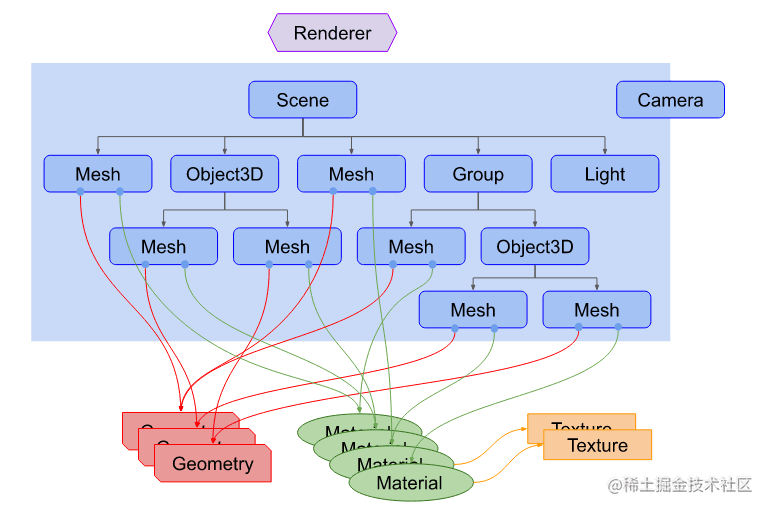（Three.js 应用架构，图片来自threejsfundamentals.org

``````<html>
<title>My first three.js app</title>
<style>
body {
margin: 0;
}
canvas {
display: block;
}
</style>
<body>
<script src="https://unpkg.com/three@0.119.0/build/three.js"></script>
<script>
//创建场景和相机
var scene = new THREE.Scene();
var camera = new THREE.PerspectiveCamera(
75,
window.innerWidth / window.innerHeight,
0.1,
1000
);

//创建渲染器，设置尺寸为窗口尺寸，并将渲染后的元素添加到body
var renderer = new THREE.WebGLRenderer();
renderer.setSize(window.innerWidth, window.innerHeight);
document.body.appendChild(renderer.domElement);

//创建一个Mesh（绿色的3D立方体），并添加到场景中
var geometry = new THREE.BoxGeometry();
var material = new THREE.MeshBasicMaterial({ color: 0x00ff00 });
var cube = new THREE.Mesh(geometry, material);

//设置照相机的位置
camera.position.z = 5;

//浏览器每次渲染的时候更新立方体的旋转角度
var animate = function () {
requestAnimationFrame(animate);

cube.rotation.x += 0.01;
cube.rotation.y += 0.01;

renderer.render(scene, camera);
};

animate();
</script>
</body>
</html>
``````# Ammo.js物理引擎

Ammo.js 是将 Bullet物理引擎 直接移植到JavaScript的产物（Bullet Physics是一个开源的物理模拟引擎）。我对物理引擎底层的工作原理理解得不太深入，简而言之，物理引擎根据你传入的参数（比如重力），创建循环，在每次循环中更新状态，从而模拟出自然的物理运动和碰撞等效果。

``````//引入库
import * as THREE from "three";
import * as Ammo from "./builds/ammo";
import {scene} from "./resources/world";

//初始化 Ammo.js 物理引擎
Ammo().then((Ammo) => {

// 创建物理世界
function createPhysicsWorld() {

//完全碰撞检测算法
let collisionConfiguration = new Ammo.btDefaultCollisionConfiguration();

// 重叠对/碰撞的调度计算
let dispatcher = new Ammo.btCollisionDispatcher(collisionConfiguration);

// 所有可能碰撞对的宽相位碰撞检测列表

// 使物体正确地交互，考虑重力、力、碰撞等
let constraintSolver = new Ammo.btSequentialImpulseConstraintSolver();

// 根据这些参数创建物理世界。 参考bullet physics文档
let physicsWorld = new Ammo.btDiscreteDynamicsWorld(
dispatcher,
overlappingPairCache,
constraintSolver,
collisionConfiguration
);

// 添加重力
physicsWorld.setGravity(new Ammo.btVector3(0, -9.8, 0));
}

//创建球体
function createBall(){
//球体参数
let pos = {x: 0, y: 0, z: 0};
let quat = {x: 0, y: 0, z: 0, w: 1};
let mass = 3;

//three.js相关代码

//创建球体并添加到场景中
let ball = new THREE.Mesh(new THREE.SphereBufferGeometry(radius), new THREE.MeshStandardMaterial({color: 0xffffff}));
ball.position.set(pos.x, pos.y, pos.z);

//Ammo.js相关代码

//设置位置和旋转
let transform = new Ammo.btTransform();
transform.setOrigin(new Ammo.btVector3(pos.x, pos.y, pos.z));
transform.setRotation(
new Ammo.btQuaternion(quat.x, quat.y, quat.z, quat.w)
);

//设置物体运动
let motionState = new Ammo.btDefaultMotionState(transform);

//设置碰撞边界框
collisionShape.setMargin(0.05);

//设置惯性
let localInertia = new Ammo.btVector3(0, 0, 0);
collisionShape.calculateLocalInertia(mass, localInertia);

//生成创建刚体（物体）的结构信息
let rigidBodyStructure = new Ammo.btRigidBodyConstructionInfo(
mass,
motionState,
collisionShape,
localInertia
);

//基于上面的结构信息创建物体
let body = new Ammo.btRigidBody(rigidBodyStructure);

//当物体运动时，为其添加摩擦力
body.setFriction(10);
body.setRollingFriction(10);

// 将物体添加到物理世界，这样Ammo.js引擎才能不断更新物体的状态
}

createPhysicsWorld();
createBall()
}
``````

# 运动和交互

``````//渲染框架
function renderFrame() {

//记录上一次渲染的时间
let deltaTime = clock.getDelta();

//基于用户输入，计算球会受到的力和产生的速度
moveBall();

//根据时间更新物理世界状态
updatePhysics(deltaTime);

//进行渲染
renderer.render(scene, camera);

// 循环
requestAnimationFrame(renderFrame);
}

//更新物理世界状态的方法定义
function updatePhysics(deltaTime) {

physicsWorld.stepSimulation(deltaTime, 10);

//遍历“刚体”列表，并更新物理世界中的所有刚体状态
for (let i = 0; i < rigidBodies.length; i++) {

//变量定义：three.js需要的meshObject，和ammo.js需要的ammoObject
let meshObject = rigidBodies[i];
let ammoObject = meshObject.userData.physicsBody;

//获取物体当前运动状态
let objectMotion = ammoObject.getMotionState();

//如果物体正在移动，则获取物体的当前位置和旋转信息
if (objectMotion) {
objectMotion.getWorldTransform(transform);
let mPosition = transform.getOrigin();
let mQuaternion = transform.getRotation();

// 更新物体的位置和旋转状态
meshObject.position.set(mPosition.x(), mPosition.y(), mPosition.z());
meshObject.quaternion.set(mQuaternion.x(), mQuaternion.y(), mQuaternion.z(), mQuaternion.w());
}
}
}
``````

# 用户输入

``````// 在坐标平面上保持对当前球体运动的跟踪
let moveDirection = { left: 0, right: 0, forward: 0, back: 0 };

//控制器div在屏幕上的位置坐标
let coordinates = { x: 0, y: 0 };

//保存触摸事件的起始坐标的变量
let dragStart = null;

//创建控制器div元素
const stick = document.createElement("div");

//监听用户触摸点的移动
function handleMove(event) {
//没有移动，返回
if (dragStart === null) return;

//有移动，获取新的触摸点的x、y坐标
if (event.changedTouches) {
event.clientX = event.changedTouches.clientX;
event.clientY = event.changedTouches.clientY;
}

//根据触摸点的移动，计算出控制器div的实时坐标
const xDiff = event.clientX - dragStart.x;
const yDiff = event.clientY - dragStart.y;
const angle = Math.atan2(yDiff, xDiff);
const distance = Math.min(maxDiff, Math.hypot(xDiff, yDiff));
const xNew = distance * Math.cos(angle);
const yNew = distance * Math.sin(angle);
coordinates = { x: xNew, y: yNew };

//根据实时坐标更新样式
stick.style.transform = `translate3d(\${xNew}px, \${yNew}px, 0px)`;

//根据坐标计算出球的运动方向
touchEvent(coordinates);
}

//根据用户的触摸点移动坐标计算出球的运动方向
function touchEvent(coordinates) {

// 向右运动
if (coordinates.x > 30) {
moveDirection.right = 1;
moveDirection.left = 0;
// 向左运动
} else if (coordinates.x < -30) {
moveDirection.left = 1;
moveDirection.right = 0;
} else {
moveDirection.right = 0;
moveDirection.left = 0;
}

//向前运动
if (coordinates.y > 30) {
moveDirection.back = 1;
moveDirection.forward = 0;
//向后运动
} else if (coordinates.y < -30) {
moveDirection.forward = 1;
moveDirection.back = 0;
} else {
moveDirection.forward = 0;
moveDirection.back = 0;
}
}
``````